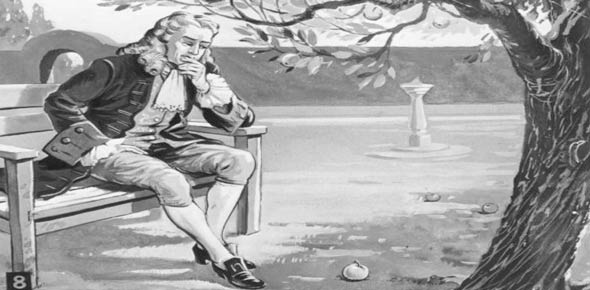# 2A651H Vol. 2

100 Questions | Total Attempts: 170Settings.

Related Topics
• 1.
"An unbalanced force on a body produces or tends to produce acceleration in the direction of the force and that the acceleration, if any, is directly proportional to the force and inversely proportional to the mass of the body." This statement is?
• A.

Bernoulli's Principle

• B.

Newton's First Law of Motion

• C.

Newton's Second Law of Motion

• D.

Newton's Third Law of Motion

• 2.
Newton's Third Law of Motion explains why an operating jet engine produces
• A.

Forward thrust

• B.

Forward acceleration

• C.

Increased acceleration

• D.

Decreased acceleration

• 3.
Which law may you sum up with the words action and reaction?
• A.

Bernoulli's Principle

• B.

Newton's First Law of Motion

• C.

Newton's Second Law of Motion

• D.

Newton's Third Law of Motion

• 4.
The temperature of compressed air in a jet engine must be raised to
• A.

Increase energy

• B.

Decrease energy

• C.

Increase volume

• D.

Decrease volume

• 5.
Which section of a jet engine keeps the compressor rotating?
• A.

Turbine

• B.

Exhaust

• C.

Diffuser

• D.

Combustion

• 6.
Through which section of a jet engine is energy expended into the atmosphere, resulting in thrust?
• A.

Turbine

• B.

Exhaust

• C.

Diffuser

• D.

Combustion

• 7.
Which type of horsepower is the power developed within the combustion chambers without reference to friction losses within the engine?
• A.

Brake

• B.

Indicated

• C.

Frictional

• D.

Equivalent shaft

• 8.
Which instrument is used to measure atmospheric pressure?
• A.

Barometer

• B.

Thermometer

• C.

Psychrometer

• D.

Potentiometer

• 9.
The standard atmospheric pressure in pounds per square inch (psi) is equal to
• A.

13.5 psi

• B.

14.7 psi

• C.

29.92 psi

• D.

40.55 psi

• 10.
The actual amount of the water vapor in a mixture of air and water is the definition of
• A.

Pressure altitude

• B.

Relative humidity

• C.

Absolute humidity

• D.

Atmospheric pressure

• 11.
The ratio of the amount of water vapor actually present in the atmosphere to the amount that would be present if the air were saturated at the prevailing temperature and pressure is the definition of
• A.

Pressure altitude

• B.

Relative humidity

• C.

Absolute humidity

• D.

Atmospheric pressure

• 12.
The major sections of a turboprop engine are the power unit, torquemeter assembly, and
• A.

Turbine assembly

• B.

Compressor assembly

• C.

Combustion assembly

• D.

Reduction Gearbox (RGB) assembly

• 13.
What is the overall reduction ratio of the reduction gearbox's (RGB) main reduction gear train?
• A.

3.125 to 1

• B.

4.333 to 1

• C.

13.54 to 1

• D.

13,820 to 1

• 14.
The four sections of the T56 engine power unit are the compressor, combustion, turbine, and
• A.

Torquemeter

• B.

Safety coupling

• C.

Reduction gearbox (RGB)

• D.

Accessory drive housing assembly

• 15.
Which compressor section assembly disperses compressor discharge air and directs it to the combustion chambers (liners)?
• A.

Diffuser

• B.

Compressor rotor

• C.

Compressor housing

• D.

Compressor air inlet housing

• 16.
During flight range operation, what maintains the T56 engine at a constant engine speed of 13,820 revolutions per minute (RPM)?
• A.

Fuel control

• B.

Electrical system

• C.

Hydraulic control system

• D.

Propeller governing system

• 17.
Which two gears make up the first stage of reduction in the reduction gearbox (RGB)?
• A.

Sun and Planetary

• B.

Ring and ring coupling

• C.

Accessory drive and starter

• D.

Pinion input and main drive

• 18.
What is the total gear reduction ratio of the reduction gearbox (RGB)?
• A.

3.125:1

• B.

4.333:1

• C.

12.85:1

• D.

13.54:1

• 19.
What is not a propeller brake position?
• A.

Locked

• B.

Applied

• C.

Engaged

• D.

Released

• 20.
When the engine is running above 23% revolutions per minute (RPM), the propeller brake is held in the position by the
• A.

Release spring

• B.

Starter gear and helical splines

• C.

Outer member and accessory drive gear

• D.

Reduction gearbox (RGB) system oil pressure

• 21.
Which position is the propeller brake in when the aircraft is on the ground and the engine is stopped?
• A.

Locked

• B.

Applied

• C.

Engaged

• D.

Released

• 22.
When the engine is operating at a throttle setting of Flight Idle or above, what preset limit of inch-pounds of torque must be exceeded in order for the negative torque signal (NTS) system to actuate the actuator rod?
• A.

-1,020 + 400

• B.

-1,140 + 500

• C.

-1,260 + 600

• D.

-1,380 + 700

• 23.
When the engine is operating below Flight Idle, the negative torque signal (NTS) system is
• A.

Mechanically locked in

• B.

Electronically locked in

• C.

Mechanically locked out

• D.

Electronically locked out

• 24.
Torque is transferred from the power unit to the safety coupling on the reduction gearbox (RGB) by the
• A.

Torque Shaft

• B.

Reference Shaft

• C.

Torquemeter Housing

• D.

Compressor Extension Shaft

• 25.
The amount of twisting deflection is determined by the
• A.

Torque Shaft

• B.

Reference Shaft

• C.

Safety Coupling

• D.

Pinion Input Gear